Courses

# Time And Work Quant Notes | EduRev

## Quant : Time And Work Quant Notes | EduRev

The document Time And Work Quant Notes | EduRev is a part of the Quant Course Quantitative Techniques for CLAT.
All you need of Quant at this link: Quant

Chapter - 18

TIME AND WORKTo solve problems on ‘time and work’ the following simple facts should be remembered.

1. If a person can do a work in 5 days, then in one day he will do 1/5th of the whole work. Conversely, if a man can do 1/5th of the work in one day, he will complete the whole work in 5 days.

2. If the number of men engaged to do a piece of work be increased in a certain ratio, the time required to do the same work will be decreased in the same ratio and vice versa. Thus if the number of men be changed in the ratio of 3 : 7, the time required will be changed in the ratio of 7 : 3.

3. If A is twice as good a workman as B than A will take one-half of the time taken by B to do a certain piece of work.

To sum up if M1 persons working H1 hours a day can do W1 work in D1 days and M2 persons working H2 hours a day can do W2 work in D2 days, then the following equation will hold good.

M1 D1 H1 W2 = M2 D2 H2 W1

SOLVED EXAMPLES

Ex. 1: A does a work in 10 days and B does the same work in 15 days. How many days they will take to do the work together.

Solution: A does the work in 10 days

So A’s one day work = 1/10

B does the work in 15 days

So B’s one day work = 1/15

Work done by A and B in one day = 1/10 + 1/15 = 5/30

= 1/6

So A and B together will be able to finish the work in 6 days.

Ex. 2: A can finish a work in 18 days and B can finish the same work in 9 days. If they work together, what part of the work will they be able to finish in a day.

Solution: A finishes the work in 18 days.

So A’s one day work = 1/18

B finishes the same work in 9 days.

So B’s one day work is 1/9.

Work done by A and B in one day = 1/18 + 1/9 = 3/18 = 1/6

Ex. 3: A tyre has two punctures. The first puncture alone can empty the tyre in 9 minutes and 2nd puncture alone can empty the tyre in 6 minutes. How long will both the punctures take to flat the tyre.

Solution: First puncture takes 9 minutes to flatten the tyre.

So first puncture’s one minute work = 1/ 9

Second puncture takes 6 minutes to flatten that tyre

So second puncture’s one minute work = 1/6

So work done by both punctures in one minute

= 1/9 + 1/6 = 5/18

Therefore both punctures together will take 18/5 minutes to flatten the tyre.

Ex 4. A man can do a job in 5 days but with the help of his son he can do it is 3 days. In what time can the son do it alone.

Solution: The man can do the job in 5 days

So his one day work = 1/5

Man and son’s one day work = 1/3

Son’s one day work = 1/3 – 1/5 =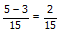So son alone can do it in 15/2 days.

Ex 5: A and B together can finish a job in 15 days. If A alone can finish the job in 25 days, in how many days can B alone finish the job.

Solutions: Time taken by A and B together to finish the job = 15 days.

So in one day they can finish 1/15 of the job. A alone can finish the job in 25 days. So A’s one day work = 1/25

B’s one day work =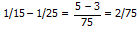So B alone can finish the job in 75/2 days.

Ex. 6: A and B together can build a house in 25 days. They work together for 15 days and then B goes away. A finishes the rest of the work in 20 days. How long will each take to finish the job working separately.

Solution: Time taken by A+B to finish the work is 25 days. So in one day (A+B) will finish 1/25 of the work. They work together for 15 day, so the work done by A+B in 15 days =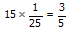Remaining job =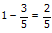A complete 2/5 of work in 20 days

A can complete one work in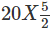= 50 days

B’s one day work = 1/25 – 1/50 = 1/50

So B can finish the whole work in 50 days.

Ex. 7: A can finish a job in 10 days, B in 12 days and C in 10 days. In how many days will they finish the job if they work together.

Solution: A’s one day work = 1/10

B’s one day work = 1/12

C’s one day work = 1/10

A+B+C’s one day work = 1/10 + 1/12 + 1/10 =

(6+5+6)/60 = 17/60

So together they can complete the work in 60/17 days.

Ex. 8: Mary alone can finish a painting job in 12 days. Gary alone can do the same job in 20 days. Mary works alone for 4 days and then Gary joins in to help. In how many days will they finish the remaining job.

Solutions: Mary’s one day work = 1/12

Gary’s one day work = 1/20

Mary works for 4 days. His work = 1/12 x 4 = 1/3

Remaining work = 1 – 1/3 = 2/3

(Gary + Mary)’s work in one day = 1/12 + 1/20 = 8/60

8/60 of the work in done by (Gary+Mary) in one day

1 of the work in done by (Gary+Mary) = 60/8

2/3 of the work in done by = 60/8 x 2/3 = 5 days

QUESTIONS ON PIPES AND CISTERNS

Ex. 9: Tap A can fill a tank in 8 hours. Outlet B can empty the tank in 12 hours. If both are kept open, how long will it take to fill the tank.

Solution: Tap A can fill the tank in 8 hours.

So Tap A’s one hour work = 1/8

Tap B can empty the tank in 12 hours

So Tap B’s one hour work = 1/12

Tap A and Tap B’s one hour work = 1/8 – 1/12 = (3 – 2) / 24

= 1/24

So the tank will be full after 24 hours.

10. Tap A and B can separately fill a tank in 10 hours and 15 hours respectively. If both the taps are opened together, how long will it take for the tank to be full.

Solution: Tap A fills the tank in 10 hours.

So Tap A’s one hour work = 1/10

Tap B fills the tank in 15 hours

Tap B’s one hour work = 1/15

Both A and B together fill 1/10 + 1/15 of tank in one hour which is equal to (3+2)/30 = 5/30 = 1/6

So both A and B will fill the tank in 6 hours.

Ex. 11: 8 Taps can fill a reservoir in 90 minutes. In how much time 12 taps can fill up the same reservoir if all the taps have equal capacity.

Solution: 8 taps fill the reservoir in 90 minutes.

One taps fill the reservoir in 90 x 8 minutes.

12 taps fill the reservoir in = (90 x 8) / 12 = 60 minutes.

Ex. 12: Pipe A can fill a tank in 3 hours. Pipe B can fill it in 4 hours. An outlet pipe C can empty the filled-in tank in 6 hours. If all the three pipes are kept open simultaneously, in how many hours will the tank be half full?

Solution: Pipe A can fill the tank in 3 hours

Pipe A will fill 1/3 tank in one hour

Pipe B can fill the tank in 4 hours.

So pipe B will fill ¼ tank in one hour

Pipe C can empty the tank in 6 hours

So in one hour pipe C will empty 1/6 of the tank

Pipe A + Pipe B + Pipe C will fill 1/3 + ¼ - 1/6 tank in one hour or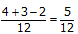So three pipes can fill the tank in 12/5 hours.

½ tank will be filled in ½ x 12/5 = 6/5 hours.

Ex. 13: Tap A fills a tank in 2 hours. Outlets B and C can empty the tank in 4 hours and 3 hours respectively. If the tank is empty and A and B are opened, how long will it take to fill the tank. After the tank is full A, B, C are all opened, in how many hours will the tank be empty.

Solution: A’s one hour work = ½ tank

B can empty ¼ of tank in one hour

C can empty 1/3 of tank in one hour

Tank is empty A+B’s one hour work = ½–1/4 = ¼

So it will take 4 hours to fill the tank

Now the tank is full. A+B+C’s one hour work

= ½ - ¼ - 1/3 = 6- 3- 4 / 12 =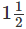So filled up tank will be emptied in 12 hours.

Offer running on EduRev: Apply code STAYHOME200 to get INR 200 off on our premium plan EduRev Infinity!

## Quantitative Techniques for CLAT

63 videos|22 docs|93 tests

,

,

,

,

,

,

,

,

,

,

,

,

,

,

,

,

,

,

,

,

,

;